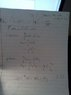# WOrk done by a variable force (in two dimension)

daivinhtran

## Homework Statement

How to find the work done by a variable force in (two dimension)

When
F = ax^2 i + b y^3 j

If a subject move from (x1,y1) to (x2, y2)

F = dW/dr

## The Attempt at a Solution

I tried to solve them separately by x-direction and y-direction, and then I added them up
like Wx + Wy = W
Am I right?

Last edited:

Gold Member
In this problem the path taken from (x1,y1) to (x2, y2) is important, different paths taken give different results. In this problem I assume the path is a straight line. If you solve the problem as you suggested I'm not sure you took into account the path taken? Can you scan your work and post it?

Hopefully others will help.

daivinhtran
In this problem the path taken from (x1,y1) to (x2, y2) is important, different paths taken give different results. In this problem I assume the path is a straight line. If you solve the problem as you suggested I'm not sure you took into account the path taken? Can you scan your work and post it?

Hopefully others will help.

https://www.physicsforums.com/attachment.php?attachmentid=51764&stc=1&d=1349922834

I hope I solve it right

#### Attachments

•IMG054.jpg
15.4 KB · Views: 1,517
Gold Member
I don't think what you did is right. You did not take the path into account only the end points. Start from the diffinition of infitesimal work, dW = vector F dot vector dr and plug and chug. You must come up with a vector expression for dr for the straight line path. You can do this as you know the endpoints.

daivinhtran
I don't think what you did is right. You did not take the path into account only the end points. Start from the diffinition of infitesimal work, dW = vector F dot vector dr and plug and chug. You must come up with a vector expression for dr for the straight line path. You can do this as you know the endpoints.

as I stated before, the particle goes from (x1,y1) to (x2, y2)
so the end point is (x2, y2)

So, am I doing this right?

P/s: however, the path between the two points is straight line

Gold Member
I don't think so. Hopefully others will confirm.